• NASCH模型、单车道CA模型编程实现
2021-12-22 14:38:05

交通流理论

NASCH模型

单车道CA模型

（本括号中内容编写于2021/12/24，交报告截止了，想要参考报告格式的可以去我的资源下载，已经上传）

PYTHON编程实现

边界：周期性边界条件

数据：扔掉前2000个时间步，对后2000个进行统计

给出了基本图

流量密度关系

时空图

分别对应NASCH_yys_1,2,3；（ps:2021/12/28之前使用下述代码交实验报告请注明代码出处）

下面对于1部分代码分享给大家，2，3有需要自取。（1运行几秒钟出结果、2、3分别要运行五六分钟左右）

先上图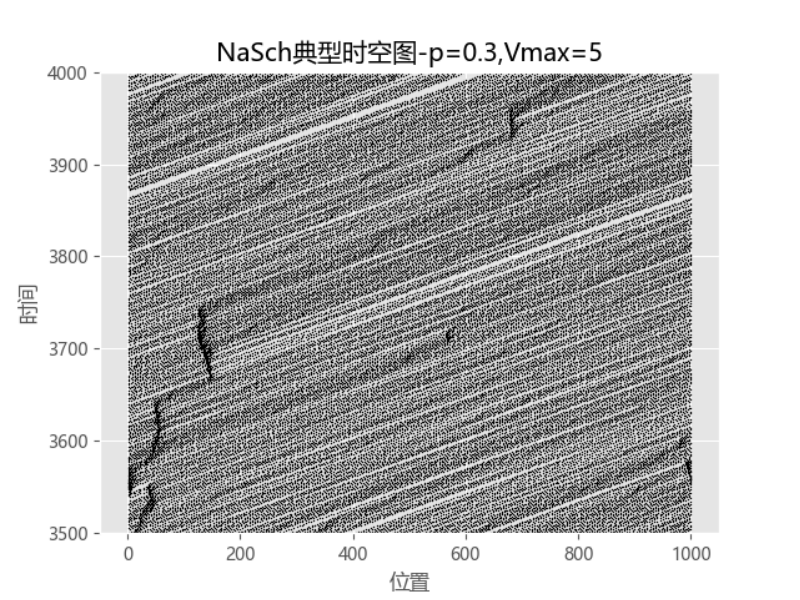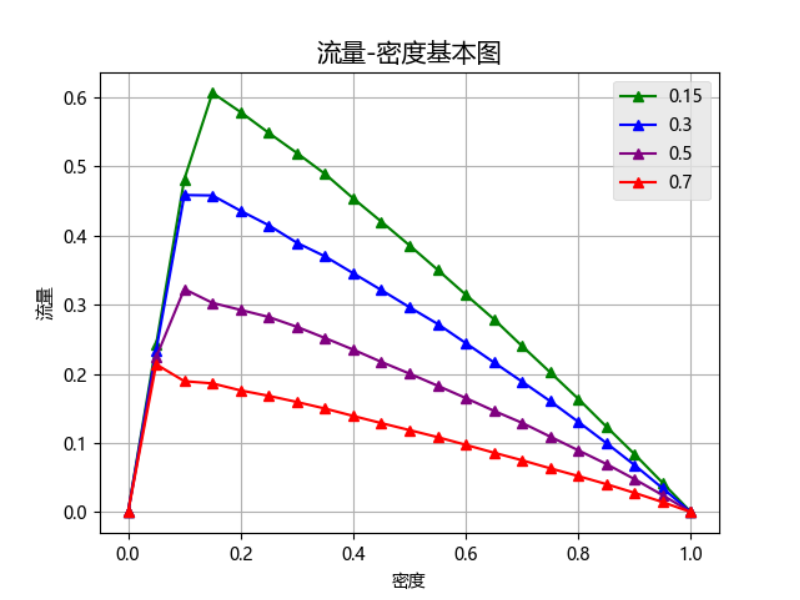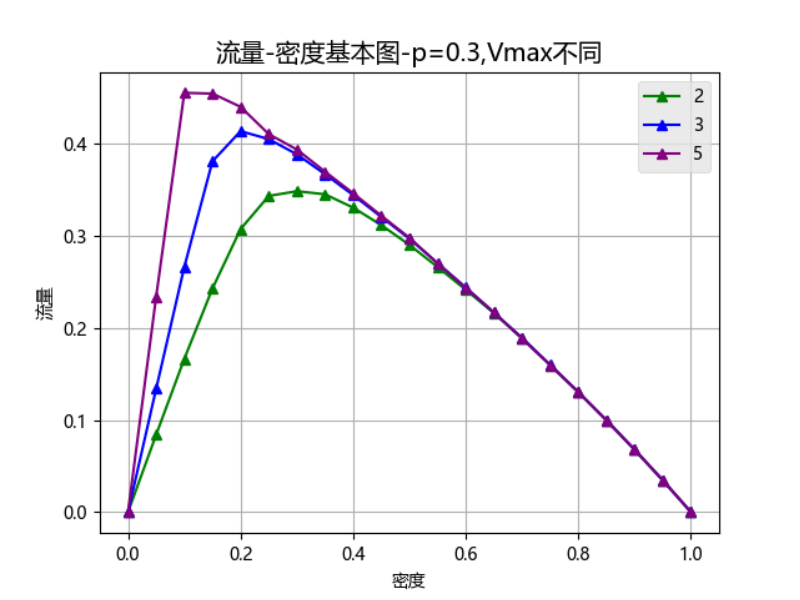解释：

1.1  代码函数详解

1.1.1  draw_time_space( )函数

此函数为自编函数，主要用于绘画时空图。

调用matplotlib库，plt.style.use( )用于设置绘图样式，axes.set_ylim( )用于设置绘图时间步区间，plt.scatter用于绘出时空图的散点，散点的颜色、大小与样式。

1.1.2  draw_volume_density(x_list, y_list,q,p )函数

此函数为自编函数，主要用于流量密度图。

具体的调用与时空图调用类似，相同的不过多赘述。plt.plot( )用于绘出折线图。

1.1.3  Speed_acceleration(a)函数

此函数为自编函数，主要用于CA模型的加速阶段。

调用系统max函数，将速度加1和速度上限的较小值赋给a并且返回这个值。

1.1.4  Speed_slowdown(a)函数

此函数为自编函数，主要用于CA模型的减速阶段。

调用系统min函数，将速度减1和车辆前方空挡数（调用d_empty(a)函数）较小值赋给a并且返回这个值。

1.1.5  Speed_random_slow(a)函数

此函数为自编函数，主要用于CA模型的随机慢化阶段。

调用系统random函数，生成0-1之间的随机浮点数，使用if语句：对于随机数小于p_slow的，进行慢化处理，取0和速度减1的较大值并返回；对于随机数大于p_slow的，保留原值并返回。

1.1.6  d_empty(a)函数

此函数为自编函数，主要用于CA模型中元胞寻找前方最大的空格数（使用周期性边界）。

使用sum_empty进行计数，进行两个循环，从位置a开始寻找，如果前方位置为None，说明前方有空格，sum_empty加1，直到前方不是None，则返回sum_empty的值。

1.1.7  initial_veh( )函数

此函数为自编函数，根据num_veh车辆数初始化元胞车道的车辆位置以及速度。

调用系统random函数，生成随机速度处于0-5，随机位置0-999，对于位置处速度为None，说明无车，则插入这辆车，直到插入的车辆数达到num_veh。

1.1.8  initial_numbers( )函数

此函数为自编函数，根据要求生成50-950辆车的数据进列表numbers_veh。

1.1.9  get_mean_speed_density(a，b)函数

此函数为自编函数，设置虚拟检测器，计算a-b段的某个密度下的车辆的平均速度，并且返回平均速度的值。（此函数亦可同时检测某段的密度）

上部分截取自我的实验报告。

下部分为代码1（如不通可能是被我删了某些函数，资源里面是完整的，供下载）

#-*-coding:GBK -*-
import matplotlib.pyplot as plt
import random
import numpy as np
import matplotlib

plt.rcParams['font.sans-serif'] = ['Microsoft YaHei']

#找空档 周期性边界
def d_empty(a):
sum_empty=0
if cell_list[j]==None:
sum_empty=sum_empty+1
else:
return sum_empty
for j in range(0,a):
if cell_list[j]==None:
sum_empty=sum_empty+1
else:
return sum_empty
#加速
def Speed_acceleration(a):
return min(a+1,Vmax)
#减速
def Speed_slowdown(a,b):
return min(a,d_empty(b))
#随机慢化
def Speed_random_slow(a):
b=random.random()
if b<=p_slow:
return max(0,a-1)
else :
return a
#位置更新
#初始化
def initial_veh():
sum1=0
while True:
a=random.randint(0,Vmax)
b=random.randint(0,999)
if cell_list[b]==None:
sum1=sum1+1
cell_list[b]=a
if sum1==num_veh:
break
#参数设置
running_time = 4000
# ~ V_max=[2,3,5]
Vmax=5
p_slow=0.3
num_veh=700

initial_veh()
cell_list1=cell_list.copy()
time=[]
space=[]
s=[]
Volume_list1=[]
for i in range(0,running_time):
if cell_list1[cell]!=None:
#调用浪费时间
#cell_list[cell]=Speed_acceleration(cell_list[cell])
#cell_list[cell]=Speed_slowdown(cell_list[cell],cell)
cell_list[cell]=min(cell_list[cell]+1,Vmax)
cell_list[cell]=min(cell_list[cell],d_empty(cell))
cell_list[cell]=Speed_random_slow(cell_list[cell])
#位置更新
h=cell_list[cell]
cell_list[cell]=None
if i >=2000:
Volume_list1.append(volume)
#print(volume,density)
if cell_list1[j]!=None:
s.append(j+1)
space.append(s.copy())
time.append([i]*num_veh)
s=[]
cell_list1=cell_list.copy()
draw_time_space()
plt.show()python
更多相关内容
• 模型参数取值：Lroad = 1000，p = 0.3，Vmax = 5。边界条件：周期性边界。输出结果：流量密度图，时空图。
• 这是一个模拟单车道交通流三要素（流量、密度、速度）之间关系的CA模型matlab
• NaSch_3模型matlab完整代码,所有的函数以及主控文档都放在了一起，打包成doc文件 2014年数模美赛交通流仿真元胞自动机代码
• 交通流NASch模型包括比较流行的单车道、双车道、三车道等模型的模拟与仿真
• 【元胞自动机】基于元胞自动机实现NaSch模型matlab源码.md
• 交通流仿真，基于元胞自动机的一维交通流模型仿真。Nasch模型
• 一、NaSch模型 二、部分代码 % 主程序： NaSch_3.m程序代码 % 单车道最大速度 3个元胞开口边界条件加速减速随机慢化 clf clear all %build the GUI %define the plot button plotbutton=uicontrol('style','...

## 一、NaSch模型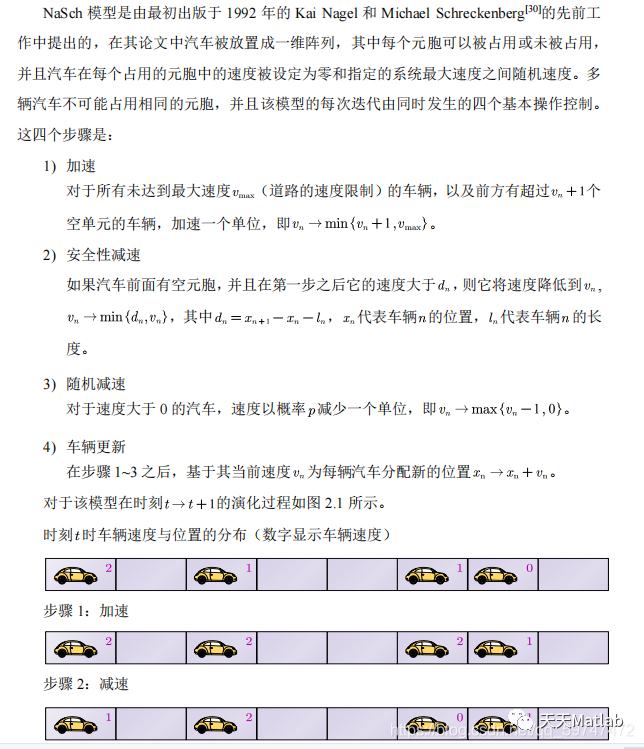## 二、部分代码

% 主程序： NaSch_3.m程序代码
% 单车道最大速度 3个元胞开口边界条件加速减速随机慢化
clf
clear all
%build the GUI
%define the plot button
plotbutton=uicontrol('style','pushbutton',...
'string','Run', ...
'fontsize',12, ...
'position',[100,400,50,20], ...
'callback', 'run=1;');
%define the stop button
erasebutton=uicontrol('style','pushbutton',...
'string','Stop', ...
'fontsize',12, ...
'position',[100,500,50,20], ...
'callback','freeze=1;');
%define the Quit button
quitbutton=uicontrol('style','pushbutton',...
'string','Quit', ...
'fontsize',12, ...
'position',[100,600,50,20], ...
'callback','stop=1;close;');
number = uicontrol('style','text', ...
'string','1', ...
'fontsize',12, ...
'position',[20,400,50,20]);
%CA setup
n=1000; %数据初始化
z=zeros(1,n); %元胞个数
cells=z;
vmax=5; %最大速度
v=speedstart(cells,vmax); %速度初始化
x=1; %记录速度和车辆位置
memor_cells=zeros(3600,n);
memor_v=zeros(3600,n);
imh=imshow(cells); %初始化图像白色有车，黑色空元胞
set(imh, 'erasemode', 'none')
axis equal
axis tight
stop=0; %wait for a quit button push
run=0; %wait for a draw
freeze=0; %wait for a freeze（冻结）
while (stop==0 & x<11502)
if(run==1)
%边界条件处理，搜素首末车，控制进出，使用开口条件
b=searchlastcar(cells);
%[cells,v]=border_control(cells,a,b,v,vmax);
for j=1:i
if i-j+1==n
continue;
else
%====================================== 加速、减速、随机慢化
if cells(i-j+1)==0; %判断当前位置是否非空
continue;
else v(i-j+1)=min(v(i-j+1)+1,vmax); %加速
%================================= 减速
k=searchfrontcar((i-j+1),cells); %搜素前方首个非空元胞位置
if k==0; %确定于前车之间的元胞数
d=n-(i-j+1);
else d=k-(i-j+1)-1;
end
v(i-j+1)=min(v(i-j+1),d);
%==============================% 减速
%随机慢化
v(i-j+1)=randslow(v(i-j+1));
new_v=v(i-j+1);
%====================================== 加速、减速、随机慢化
%更新车辆位置
z(i-j+1)=0;
z(i-j+1+new_v)=1;
%更新速度
v(i-j+1)=0;
v(i-j+1+new_v)=new_v;
end
end
end
cells=z;
memor_cells(x,:)=cells; %记录速度和车辆位置
memor_v(x,:)=v;
x=x+1;
set(imh,'cdata',cells) %更新图像
%update the step number diaplay
pause(0.0001);
stepnumber = 1+str2num(get(number,'string'));
set(number,'string',num2str(stepnumber))
end
if (freeze==1)
run = 0;
freeze = 0;
end
drawnow
end
%% NaSch时空图
figure(1)
set(gcf,'outerposition',get(0,'screensize'));
for l=1:1:3600
for k=1:1:1000
if memor_cells(l,k)> 0
plot(k,l,'k.');
hold on;
end
end
end
xlabel('空间位置 ')
ylabel('时间（s）')
set(gca,'FontSize',24,'Fontname', 'Times New Roman');
set(get(gca,'XLabel'),'Fontsize',24,'Fontname', '宋体');
set(get(gca,'YLabel'),'Fontsize',24,'Fontname', '宋体');

set(gca,'looseInset',[0 0 0 0]);
print(1,'-dpng','-r300','NaSch时空图');
%%  NaSch密度流量图
figure(2)
set(gcf,'outerposition',get(0,'screensize'));
for i=1:1:500
density(i)=sum(memor_cells(i,:)>0)/1000;
flow(i)=sum(memor_v(i,:))/1000;
end
h= plot(density,flow,'k.');
set(h,'MarkerSize',20);
%title('流量密度图 ')
xlabel('车流密度')
ylabel('车流速度')
set(gca,'FontSize',24,'Fontname', 'Times New Roman');
set(get(gca,'XLabel'),'Fontsize',24,'Fontname', '宋体');
set(get(gca,'YLabel'),'Fontsize',24,'Fontname', '宋体');
set(gca,'looseInset',[0 0 0 0]);
print(2,'-dpng','-r300','NaSch密度流量图');


## 三、仿真结果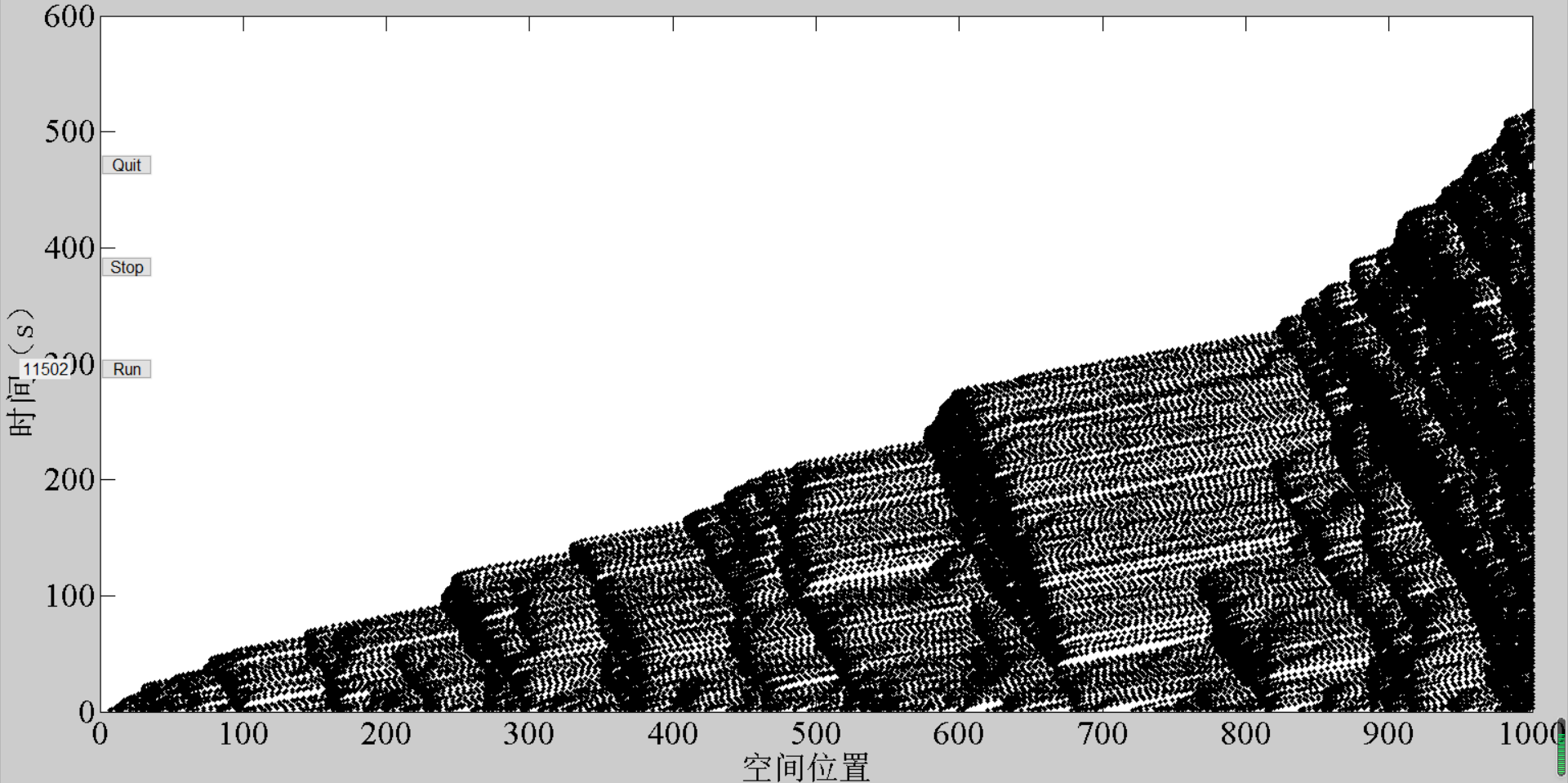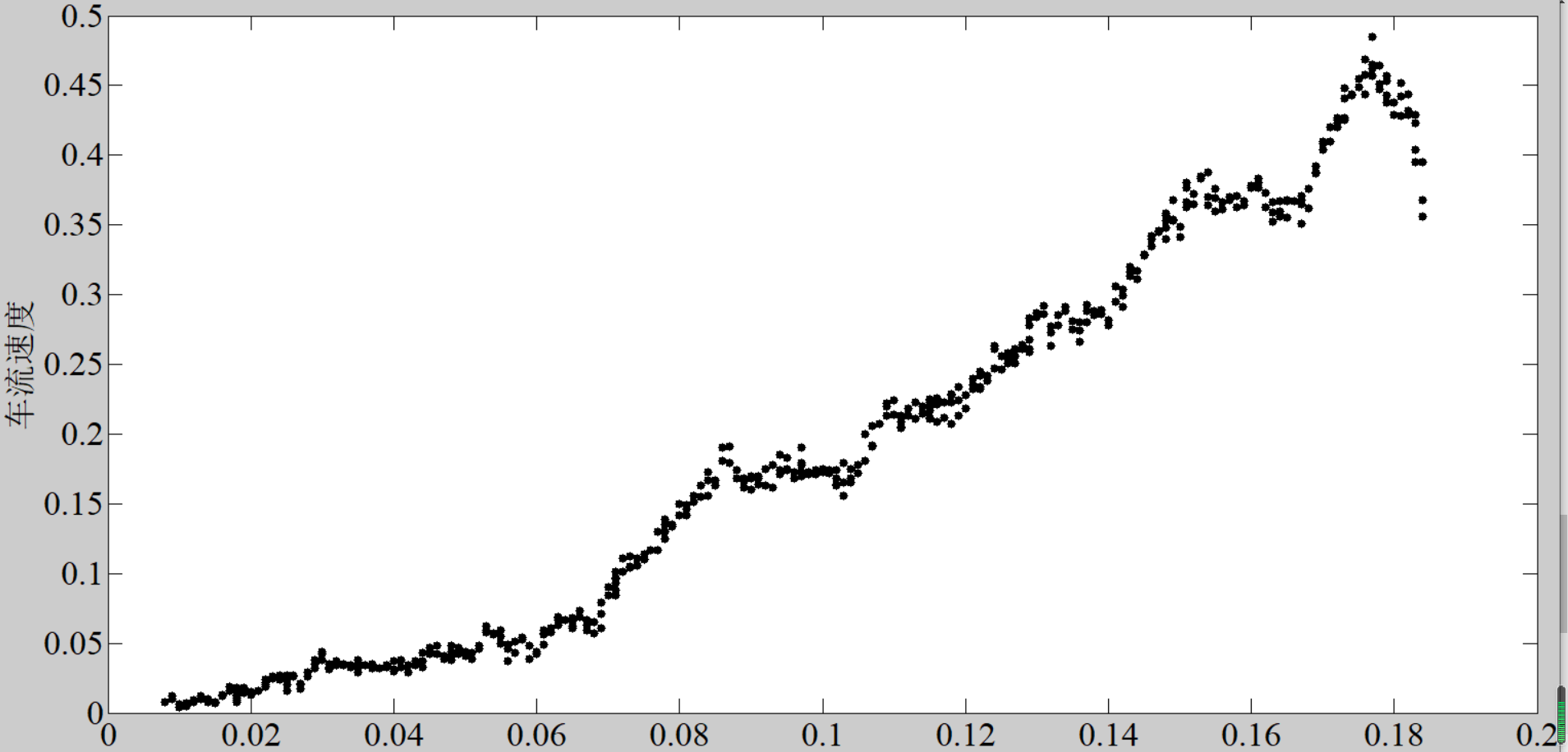展开全文• matlab 程序源代码 可用于2014年美国大学生数学建模竞赛A题
• NaSch 模型是由最初出版于 1992 年的 Kai Nagel 和 Michael Schreckenberg的先前工作中提出的，在其论文中汽车被放置成一维阵列，其中每个元胞可以被占用或未被占用，并且汽车在每个占用的元胞中的速度被设定为...

## 1 简介

NaSch 模型是由最初出版于 1992 年的 Kai Nagel 和 Michael Schreckenberg的先前工作中提出的，在其论文中汽车被放置成一维阵列，其中每个元胞可以被占用或未被占用，并且汽车在每个占用的元胞中的速度被设定为零和指定的系统最大速度之间随机速度。多辆汽车不可能占用相同的元胞，并且该模型的每次迭代由同时发生的四个基本操作控制。这四个步骤是：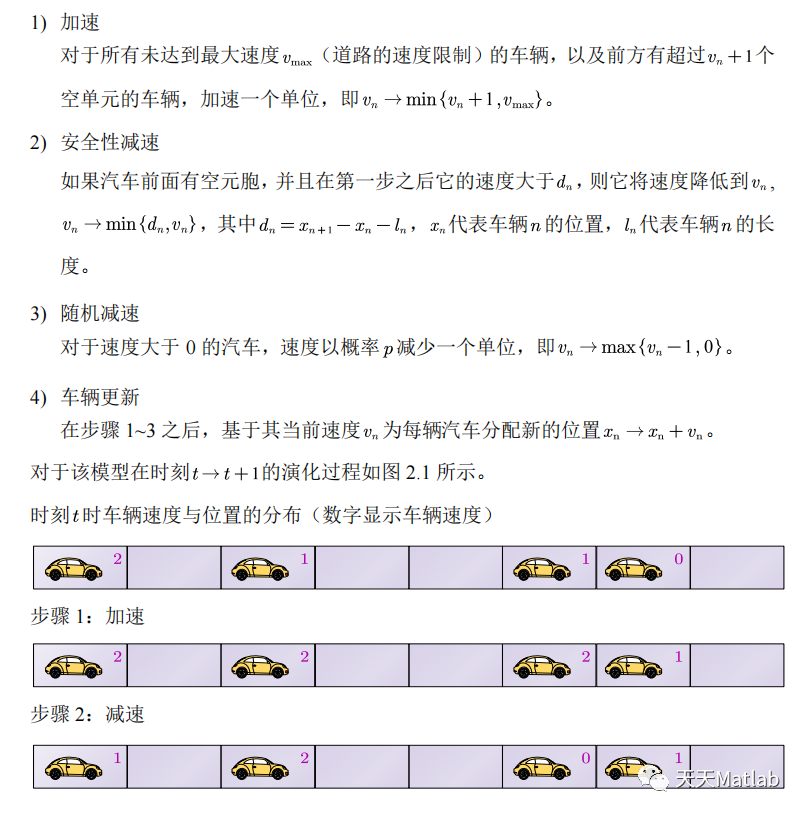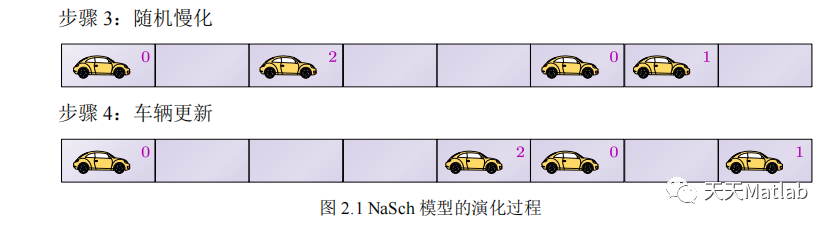## 2 部分代码

% 主程序： NaSch_3.m程序代码% 单车道最大速度 3个元胞开口边界条件加速减速随机慢化clfclear all%build the GUI%define the plot buttonplotbutton=uicontrol('style','pushbutton',...'string','Run', ...'fontsize',12, ...'position',[100,400,50,20], ...'callback', 'run=1;');%define the stop buttonerasebutton=uicontrol('style','pushbutton',...'string','Stop', ...'fontsize',12, ...'position',[100,500,50,20], ...'callback','freeze=1;');%define the Quit buttonquitbutton=uicontrol('style','pushbutton',...'string','Quit', ...'fontsize',12, ...'position',[100,600,50,20], ...'callback','stop=1;close;');number = uicontrol('style','text', ...'string','1', ...'fontsize',12, ...'position',[20,400,50,20]);%CA setupn=1000; %数据初始化z=zeros(1,n); %元胞个数z=roadstart(z,200); %道路状态初始化，路段上随机分布 200 辆cells=z;vmax=5; %最大速度​new_v=v(i-j+1);%====================================== 加速、减速、随机慢化%更新车辆位置z(i-j+1)=0;z(i-j+1+new_v)=1;%更新速度v(i-j+1)=0;v(i-j+1+new_v)=new_v;endendendcells=z;memor_cells(x,:)=cells; %记录速度和车辆位置memor_v(x,:)=v;x=x+1;set(imh,'cdata',cells) %更新图像%update the step number diaplaypause(0.0001);stepnumber = 1+str2num(get(number,'string'));set(number,'string',num2str(stepnumber))endif (freeze==1)run = 0;freeze = 0;enddrawnowend%% NaSch时空图figure(1)set(gcf,'outerposition',get(0,'screensize'));for l=1:1:3600for k=1:1:1000if memor_cells(l,k)> 0plot(k,l,'k.');hold on;endendendxlabel('空间位置 ')ylabel('时间（s）')set(gca,'FontSize',24,'Fontname', 'Times New Roman');set(get(gca,'XLabel'),'Fontsize',24,'Fontname', '宋体');set(get(gca,'YLabel'),'Fontsize',24,'Fontname', '宋体');​set(gca,'looseInset',[0 0 0 0]);print(1,'-dpng','-r300','NaSch时空图');%%  NaSch密度流量图figure(2)set(gcf,'outerposition',get(0,'screensize'));for i=1:1:500density(i)=sum(memor_cells(i,:)>0)/1000;flow(i)=sum(memor_v(i,:))/1000;endh= plot(density,flow,'k.');set(h,'MarkerSize',20);%title('流量密度图 ')xlabel('车流密度')ylabel('车流速度')set(gca,'FontSize',24,'Fontname', 'Times New Roman');set(get(gca,'XLabel'),'Fontsize',24,'Fontname', '宋体');set(get(gca,'YLabel'),'Fontsize',24,'Fontname', '宋体');set(gca,'looseInset',[0 0 0 0]);print(2,'-dpng','-r300','NaSch密度流量图');​

## 3 仿真结果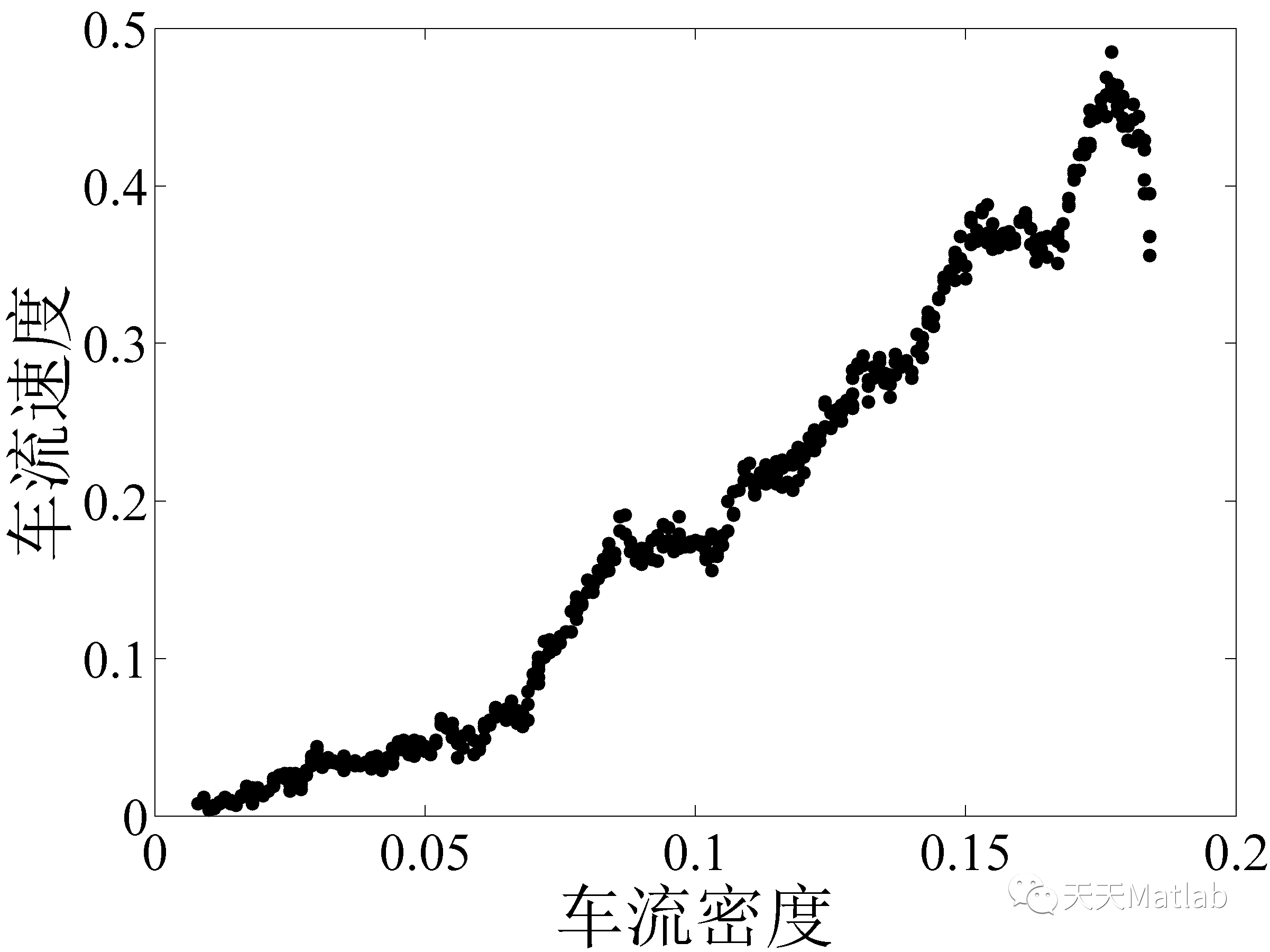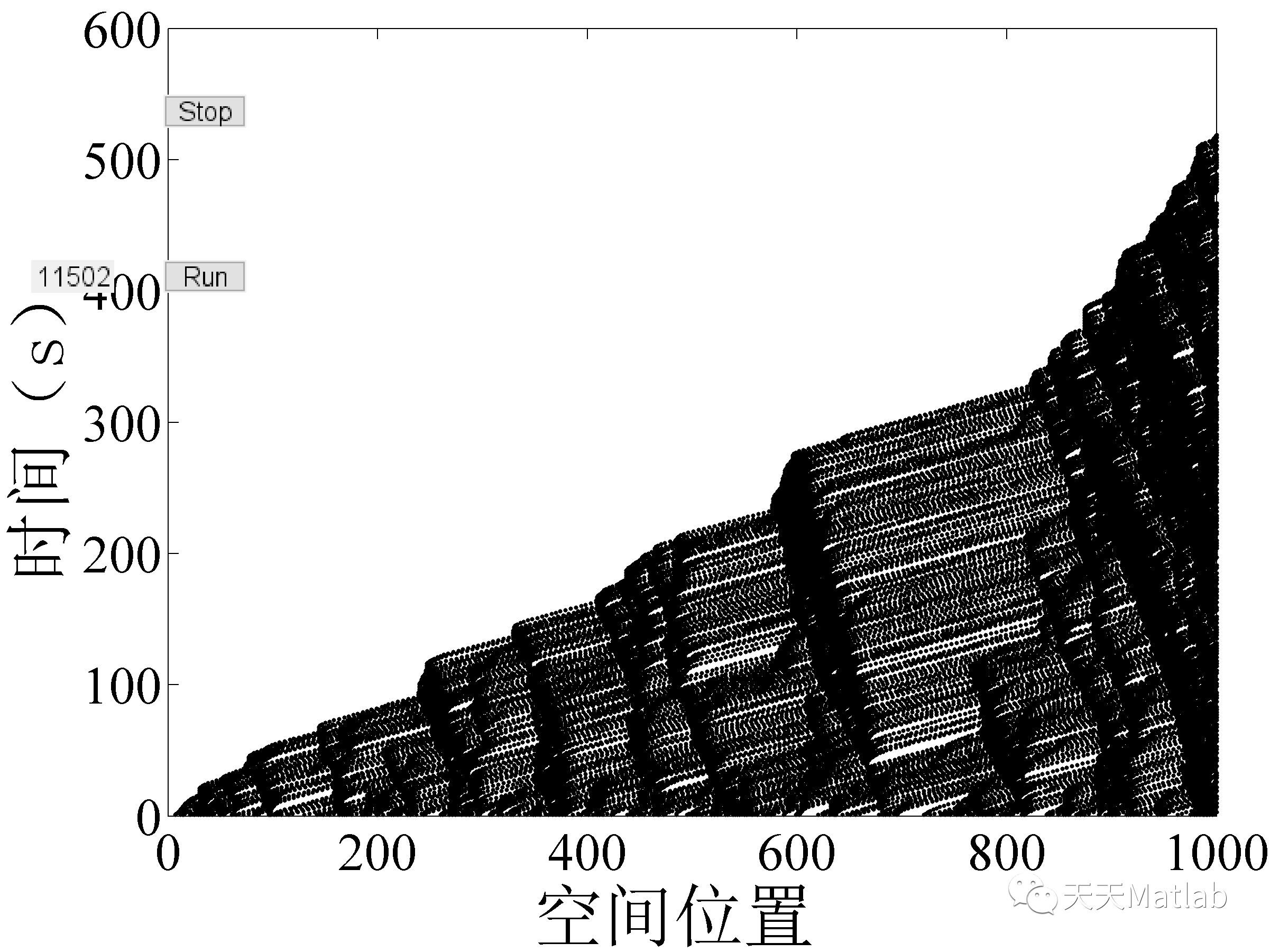## 4 参考文献

李伟娟. (2015). 基于元胞自动机的城市车辆换道模型仿真研究. 吉林大学.

### 博主简介：擅长智能优化算法、神经网络预测、信号处理、元胞自动机、图像处理、路径规划、无人机等多种领域的Matlab仿真，相关matlab代码问题可私信交流。

部分理论引用网络文献，若有侵权联系博主删除。

展开全文matlab 开发语言
• Nasch-封闭十字路口的交通流模型 nagel-schreckenberg model，模拟车流，计算平均车流量和平均速度 一位学生花钱让写的代码被骗后怒而分享源码
• 简单几步实现NaSch模型的Python仿真 NaSch模型是对184号模型的推广，1992年Nagle和Schreckenberg提出了著名的NaSch模型，在这一模型中，时间、空间以及速度都被离散化，道路被划分为离散的格子（即元胞），每个元胞...

# 简单几步实现NaSch模型的Python仿真

NaSch模型是对184号模型的推广，1992年Nagle和Schreckenberg提出了著名的NaSch模型，在这一模型中，时间、空间以及速度都被离散化，道路被划分为离散的格子（即元胞），每个元胞都是空的，或者被一辆车占据，每辆车的速度可以取1，2，…，Vmax ，Vmax 为最大速度。在时间步增加的过程中，模型按照如下规则进行演化。

#### 1 加速

Vn —> min(Vn+1, Vmax)，直观上的解释是若能加速则速度加一，反应了司机倾向于以尽可能大的速度行驶的特点。

#### 2 减速

Vn —> min(Vn, Dn)，以确保车辆不会与前车发生碰撞。

#### 3 随机慢化

以随机概率p令Vn —> max(Vn-1,0)，该规则用来体现驾驶人的行为差异，这样既可以反映随机加速行为，又可以反映减速过程中的过度反应行为。这一规则也是堵塞自发产生的至关重要因素。

#### 4 位置更新

Xn —> Xn+Vn，车辆按照更新后的速度继续向前移动。

## NaSch模型的Python实现

用python编写一个NaSch模型代码，并用Matplotlib可视化地绘制成图，并让图像随时间步的推移动态变化。

• 老规矩，先调包，并创建一个图像
import matplotlib as mpl
import matplotlib.pyplot as plt
import random
# 创建图像
fig = plt.figure(figsize=(10,1))

• 设置模型的参数，相当于一个遥控器，哪里不对点哪里~
# 模型参数设置
numofcell = 20     # 道路长度
numofcar = 12      # 空间中的车辆数
max_time = 100     # 设置最大时间步
max_speed = 5      # 允许的车辆最大速度
p_slowdown = 0.3   # 随机慢化概率
pause_time = 0.1   # 刷新时间（每一帧持续的时间）
cell_size = 15     # 元胞的大小

• 定义一个函数来构建一维空间，空间的长度就是道路长度，相当于用一系列和X轴或Y轴平行的直线，绘制一排小网格，每个小网格的中心，相当于(i,0)，其中，i=1,2,…,numofcell
# 函数：构建一维空间
def Plot_Space():
for i in range(1, numofcell): plt.plot([i-0.5, i-0.5], [-0.5, 0.5], '-k', linewidth = 0.5)
plt.axis([-0.5, numofcell-0.5, -0.5, 0.5])
plt.xticks([]);plt.yticks([])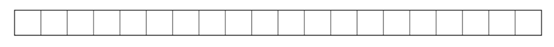• 定义一个函数来获取和前车的距离，从而避免两车相撞。在这里采用了周期性边界，即从道路末端驶出的小车会重新回到起点，相当于一个环路。
# 函数：获取和前车的距离
num = 0; i = 1
while (link2[indexofcell + i]) == None:
num += 1; i += 1
return num

# 随机生成初始元胞
Plot_Space()
num = 0
while num != numofcar:
sj = random.randint(0, numofcell - 1)
num += 1

• 在0~max_time的时间步内，分四个步骤进行NaSch模型的演化。
# NaSch模型
for t in range(0, max_time):
for cell in range(0, numofcell):
# 加速
# 减速
# 随机慢化
if random.random() <= p_slowdown:
# 位置更新
for cell in range(0, numofcell):
if new_index >= numofcell:
new_index -= numofcell

• 绘制当前时间步车辆位置的图像，注意这里有缩进，说明上一段NaSch模型演化代码中的for循环还没有结束呦~
	x1 = []
x1.append(i)
Plot_Space()
plt.plot(x1,  * numofcar, 'sk', markersize=cell_size)
plt.xlabel('timestep:' + str(t))

• 让图片动起来（同样注意缩进），plt.pause()函数让当前的图像维持pause_time长的时间，随后plt.cla()函数将整个图像擦除。下一个时间步，继续绘制图像，保留一段时间，并擦除，再绘制图像……就能源源不断地产生动画啦！
	plt.pause(pause_time)
plt.cla()


跑一下看看结果！
仔细看，拥堵带是逐渐向后传播的。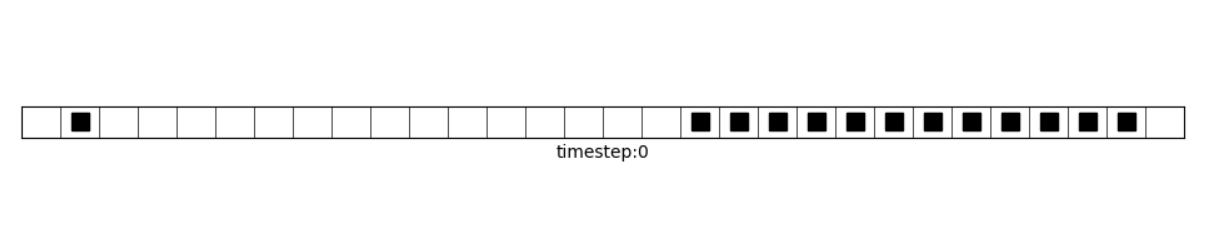操控我的“遥控器”，并给它一个恰当初始状态，元胞就可以开启“阅兵模式”。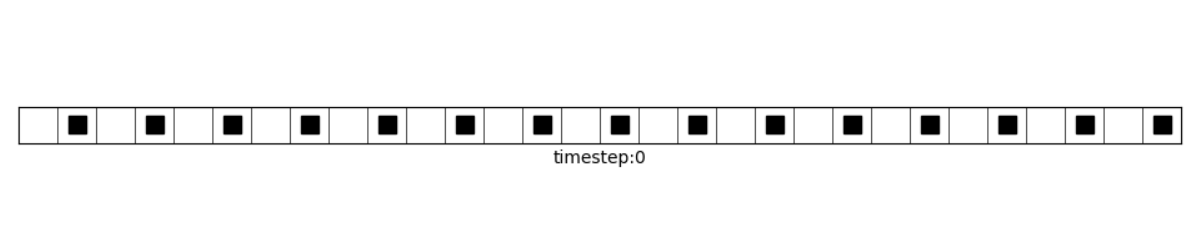给我开“瞬移”挂，只要动的足够快，你就分不清元胞到底是往左跑还是往右跑。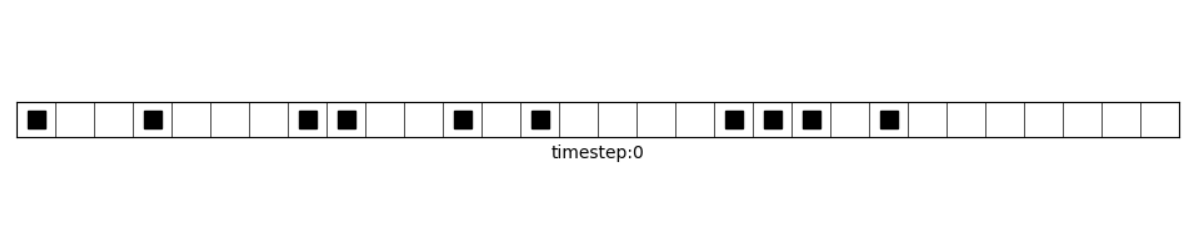以上就是全部内容啦~
WT酱的所有文章首发于公众号【交通科研Lab】~
获取更多知识请移步观看！

展开全文元胞自动机 Python编程 交通流理论
• 元胞自动机 NaSch模型及其MATLAB代码 作业要求 根据前面的介绍对NaSch模型编程并进行数值模拟 模型参数取值Lroad=1000p=0.3Vmax=5 边界条件周期性边界 数据统计扔掉前50000个时间步对后50000个时间步进行统计,需给出...
• 在分析 Origin C语言体系结构及 OriginPro辅助开发工具的基础上,针对交通流中经典的 NaSch模型进行了系统仿真 的二次开发。应用 Origin C设计了模型中的主要类,借助 OriginPro对象开发了模型的界面输入和输出函数,...
• 交通流中的NaSch双车道模型及MATLAB代码，元胞自动机。
• 元胞自动机NaSch模型MATLAB代码,元胞自动机模型步骤,matlab源码
• 交通流中的NaSch模型及MATLAB代码元胞自动机.docx
• 交通流中的NaSch模型与MATLAB代码元胞自动机.docx文档
• 交通流中的NaSch模型及MATLAB代码元胞自动机完整.pdf
• 本程序根据1992年Nagel和Schreckenberg发表的交通流论文，利用matlab编写。采用周期性边界条件，希望有所帮助